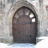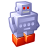# Import rule file and override the attributes

536
2
05-13-2014 05:17 AMNew Contributor
Hi,
I want to import a rule file (import.cga) to my main file (main.cga).
All parts of the import process are working well, but surprisingly the attribute values that are defined in both rules are not overridden with the values from the main file.
Could you, please, somebody tell we, where is the error? Because my final shape height is 100; and I would like to have it 10.

main file code:

` #main.cga  attr height = 10  import imp : "import.cga"  Lot-->     imp.Lot `

importing file code:

`#import.cga  attr height = 100  Lot -->  extrude(height)`
Tags (3)
1 Solution

Accepted SolutionsFrequent Contributor II
Hi !

I assume you use CE2013.1, right ?

There were some import bugs in CE2013.

Try:

`import imp : "import.cga" (height = height)`

the first height is the imported rule's attr value, which then is set to the main rule's value (2nd value).

This should work.

matt
2 RepliesFrequent Contributor II
Hi !

I assume you use CE2013.1, right ?

There were some import bugs in CE2013.

Try:

`import imp : "import.cga" (height = height)`

the first height is the imported rule's attr value, which then is set to the main rule's value (2nd value).

This should work.

mattNew Contributor
Hi Mat!

Thank you for your fast answer, yes, I am using CE2013.1. And, yes, your solution works perfectly.

Thank you very much! :)

Mary